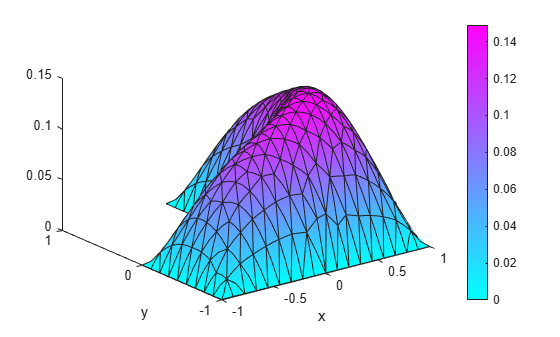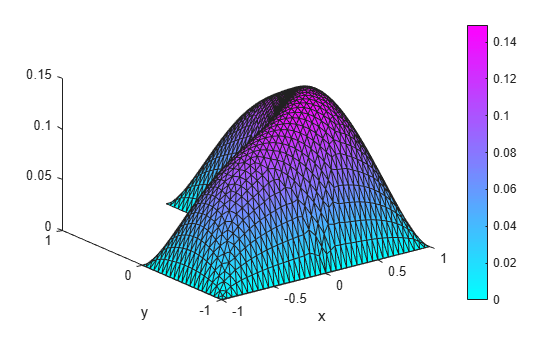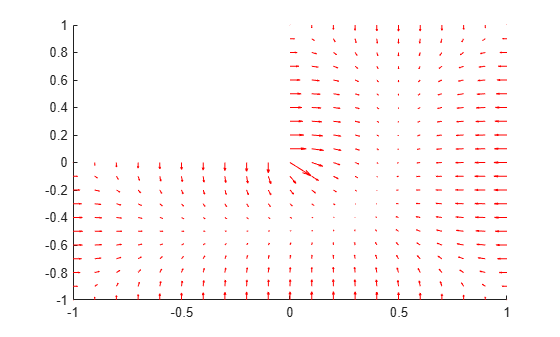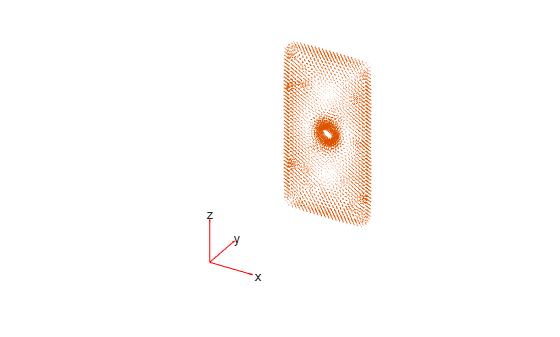# Solution and Gradient Plots with `pdeplot` and `pdeplot3D`

### 2-D Solution and Gradient Plots

To visualize a 2-D scalar PDE solution, you can use the `pdeplot` function. This function lets you plot the solution without explicitly interpolating the solution. For example, solve the scalar elliptic problem $-\nabla u=1$ on the L-shaped membrane with zero Dirichlet boundary conditions and plot the solution.

Create the PDE model, 2-D geometry, and mesh. Specify boundary conditions and coefficients. Solve the PDE problem.

```model = createpde; geometryFromEdges(model,@lshapeg); applyBoundaryCondition(model,'dirichlet', ... 'Edge',1:model.Geometry.NumEdges, ... 'u',0); c = 1; a = 0; f = 1; specifyCoefficients(model,'m',0,'d',0,'c',c,'a',a,'f',f); generateMesh(model); results = solvepde(model);```

Use `pdeplot` to plot the solution.

```u = results.NodalSolution; pdeplot(model,'XYData',u,'ZData',u,'Mesh','on') xlabel('x') ylabel('y')```To get a smoother solution surface, specify the maximum size of the mesh triangles by using the `Hmax` argument. Then solve the PDE problem using this new mesh, and plot the solution again.

```generateMesh(model,'Hmax',0.05); results = solvepde(model); u = results.NodalSolution; pdeplot(model,'XYData',u,'ZData',u,'Mesh','on') xlabel('x') ylabel('y')```Access the gradient of the solution at the nodal locations.

```ux = results.XGradients; uy = results.YGradients;```

Plot the gradient as a quiver plot.

`pdeplot(model,'FlowData',[ux,uy])`### 3-D Surface and Gradient Plots

Obtain a surface plot of a solution with 3-D geometry and N > 1.

First, import a tetrahedral geometry to a model with N = 2 equations and view its faces.

```model = createpde(2); importGeometry(model,'Tetrahedron.stl'); pdegplot(model,'FaceLabels','on','FaceAlpha',0.5) view(-40,24)```Create a problem with zero Dirichlet boundary conditions on face 4.

`applyBoundaryCondition(model,'dirichlet','Face',4,'u',[0,0]);`

Create coefficients for the problem, where `f = [1;10]` and `c` is a symmetric matrix in 6N form.

```f = [1;10]; a = 0; c = [2;0;4;1;3;8;1;0;2;1;2;4]; specifyCoefficients(model,'m',0,'d',0,'c',c,'a',a,'f',f);```

Create a mesh for the solution.

`generateMesh(model,'Hmax',20);`

Solve the problem.

```results = solvepde(model); u = results.NodalSolution;```

Plot the two components of the solution.

```pdeplot3D(model,'ColorMapData',u(:,1)) view(-175,4) title('u(1)')``````figure pdeplot3D(model,'ColorMapData',u(:,2)) view(-175,4) title('u(2)')```Compute the flux of the solution and plot the results for both components.

```[cgradx,cgrady,cgradz] = evaluateCGradient(results); figure pdeplot3D(model,'FlowData',[cgradx(:,1) cgrady(:,1) cgradz(:,1)])``````figure pdeplot3D(model,'FlowData',[cgradx(:,2) cgrady(:,2) cgradz(:,2)])```## SupportGet trial now